# Solution assignment 01 Tangent line to graph

### Assignment 1

Calculate the slope of the tangent line at the point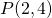of the graph of the function: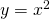### Solution

The derivative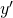of the function is: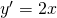At the point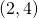the derivative has the value: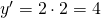and thus the slope of the tangent line atequals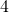.